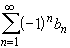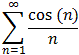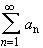#Interactive Real Analysis

Next | Previous | Glossary | Map

## 4.2. Convergence Tests

### Alternating Series Test

A series of the formwith b n0 is called Alternating Series. If the sequenceis decreasing and converges to zero, then the sum converges.
This test does not prove absolute convergence. In fact, when checking for absolute convergence the term 'alternating series' is meaningless.

It is important that the series truly alternates, that is each positive term is followed by a negative one, and visa versa. If that is not the case, the alternating series test does not apply (while Abel's Test may still work).

 Examples 4.2.20:Does the sumconverge or diverge ?Does the Alternating Series test apply to?
Proof:

Let a n = (-1) n. Then the formal sumhas bounded partial sums (although the sum does not itself converge. Why not ?). Then, with the given choice ofAbel's test applies directly, showing that the series indeed converges.Next | Previous | Glossary | Map